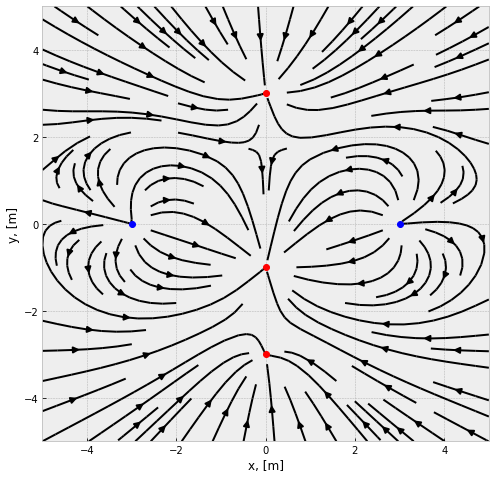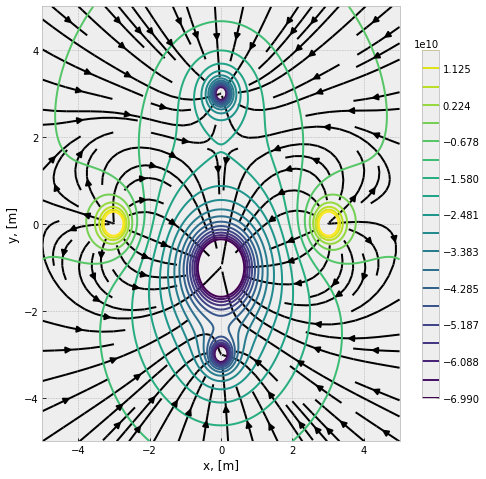# Electric fields and potentials from point charges¶

### Examples - Electromagnetism¶

Last edited: March 22nd 2018

In this notebook we will visualize the electric fields and potentials surrounding point charges. We will use among others NumPy's meshgrid function mgrid and Matplotlib's functions streamplot and contourplot for the visualizations.

### Coulomb's law¶

Coulombs law tells us that the force between two point charges are given by

$$\vec F = k_e \frac{q_1q_2}{r^2}\hat r,$$

where $k_e$ is Coulomb's constant, $q_1$ and $q_2$ are the magnitudes of the point charges, and $r$ is the distance between the charges. Coulomb's constant is often expressed as $k_e=\frac{1}{4\pi\varepsilon_0}\approx 8.988\cdot 10^9$ Nm$^2$/C$^2$, where $\varepsilon_0$ is the the vacuum permittivity.

### Electric field¶

Assume that we have an electrically charged body $A$ at a given position in space. If we now put a test charge $q_0$ at a position $P$ we will measure a force $\vec F_0$ exerted by $A$ on the charge. If the test charge is removed and replaced by a charge $q_1$ this new charge will experience a force $\frac{\vec F_0}{q_0}q_1$. This means that one can regard the force exerted on such a test charge as a property of the given potision in space created by $A$. We call this the electric field $\vec E$. In other words, the electric force on a charged body is exerted by the electric field created by other charged bodies . We define the electric field as

$$\vec E = \frac{\vec F_0}{q_0}.$$

For a point charge we get

$$\vec E= k_e \frac{q_1}{r^2}\hat r.$$

### Superposition of charges¶

Note that the superposition of forces holds for Coulomb's law, which means that the force and electric field from two (or more) point charges is the sum of the force or electric field of the charges. Mathematically, we may write the force exerted on a charge $q$ from a set of charges $\{q_i\}$ as

$$\vec F_{tot} = \sum_i \vec F_i = k_eq\sum_i \frac{q_i}{r_i^2}\hat r_i,$$

where $r_i$ is the distance between the charge $q$ and $q_i$. In the same manner, we have

$$\vec E = \sum_i\vec E_i=k_e\sum_i \frac{q_i}{r_i^2}\hat r_i.$$

If we go from the sum to an integral, we can describe continuous charge distributions, but this is not discussed in this notebook.

As always, we start by importing packages and setting common figure parameters.

In :
import numpy as np
from matplotlib import pyplot as plt
plt.style.use('bmh')


### Calculation and visualization of electric fields¶

Now, we often want to visualize the electric field around some kind of charge distribution. This is done using electric field lines, which shows the direction of $\vec E$ at each point. Their spacing can give a general idea of the magnitude $|\vec E|$. One can also express the magnitude as colors, thicknesses of the field lines or length of arrows. The field lines is often difficult or impossible to draw or calculate exact. This is where computational physics comes in handy!

Let us create a code snippet which calculates and plots the electric field around an arbitrary number of charges with an arbitrary charge. For simplicity, let us do this in two dimensions.

In :
plt.figure(figsize=(8, 8))

# Define two positive and three negative
# charges (x [m], y [m], q [C])
C = [(-3,0,1), (3,0,1), (0,3,-1), (0,-3,-1), (0,-1,-5)]

[xmin, xmax, ymin, ymax] = [-5, 5, -5, 5]
k = 8.99*10**9  # [Nm^2/C^2], in Coulomb's law

# Plot the point charges
for i in range(0, len(C)):
if C[i] > 0:
color = 'bo'
elif C[i] < 0:
color = 'ro'
else:
color = 'wo'
plt.plot(C[i], C[i], color)
plt.axis([xmin, xmax, ymin, ymax])

# Calculate the field lines
n = 200j  # Mesh grid resolution
Y, X = np.mgrid[xmin:xmax:n, ymin:ymax:n]  # Meshgrid
Ex, Ey = np.array(X*0), np.array(Y*0)
for i in range(0, len(C)):
R = np.sqrt((X-C[i])**2 + (Y-C[i])**2)
Ex = Ex + k*C[i]/R**2*(X-C[i])/R
Ey = Ey + k*C[i]/R**2*(Y-C[i])/R

# Plot the result
plt.streamplot(X, Y, Ex, Ey, color='k', density=1,
arrowstyle='simple')
plt.xlabel('x, [m]')
plt.ylabel('y, [m]')
plt.show()### Electric potentials¶

The potential energy $U$ needed to move an electric charge $q_0$ from a position $a$ to a position $b$ in a given electric field $\vec{E}$ is

$$U_{ab}=\int_a^b \vec F \cdot \text{d}\vec l = \int_a^bq_0 \vec E\cdot \text d \vec l.$$

As before, we divide by the test charge $q_0$ and get a scalar quantity which is independent of the test charge. We call this the electric potential $V$, and it's change is given by

$$\Delta V = V_a-V_b = \int_a^b \vec E\cdot \text d\vec l.$$

Often, a force infinitely far away is zero, $F_\infty=0$, and the potential $V_\infty$ here is therefore often chosen as a reference point and defined to be zero, $V_\infty\equiv 0$. This also gives the relation

$$\vec E = -\nabla V = - \left(\frac{\partial V}{\partial x}, \frac{\partial V}{\partial y}, \frac{\partial V}{\partial z}\right).$$

This means that a curve of a given potential $V(x,y,z)=\xi$, a so-called equipotential surface, is normal to the electric field lines.

In the discrete case, the electric potential due to a collection of point charges is given by

$$V=\frac{U}{q_0}=k_e\sum_i\frac{q_i}{r_i}.$$

It is easier to calculate an electric field from a potential than the other way around.

The potential can for example be visualized as equipotential surfaces (or curves in two dimensions) in different colors and/or with a given potential difference between each surface. A two dimensional potential can also be visualized in three dimensions.

Let us perform the same calculations as above, but this time calculate the electric field from the potential, $\vec E = -\nabla V$, and plot them together.

In :
plt.figure(figsize=(8, 8))

V = 0*X

# Calculate the potential
for i in range(0, len(C)):
R = np.sqrt((X-C[i])**2 + (Y-C[i])**2)
V = V + k*C[i]/R

# Calculate the electric field from the potential

# The values of the equipotential surfaces.
# Note that the potential goes to pluss or minus infinite near the point
# charges. We therefore have to exclude the maximum and minimum values.
equip_surf = np.linspace(np.min(V)*0.05, np.max(V)*0.05, 20)

# Plot the result
plt.streamplot(X, Y, Ex, Ey, color='k', density=1, arrowstyle='simple')
contour_surf = plt.contour(X, Y, V, equip_surf)
plt.colorbar(contour_surf, shrink=0.8, extend='both')
plt.xlabel('x, [m]')
plt.ylabel('y, [m]')
plt.show()### More plotting techniques¶

There are many ways to visualize fields and potentials. In this notebook we used a streamplot for the electric field and a contour plot for the electric potential. One may for example use an arrow plot to visualize a vector field and color, thickness, length or density to visualize the magnitude. The potential could also be visualized in a 3D surface plot or in a 2D plot with different colours (check out Matplotlib's function imshow). See a notebook on plotting at numfys.net or search the internet for more information!

 Young and Freedman: University Physics with Modern Physics Volume Two, 13th edition, Pearson 2011.